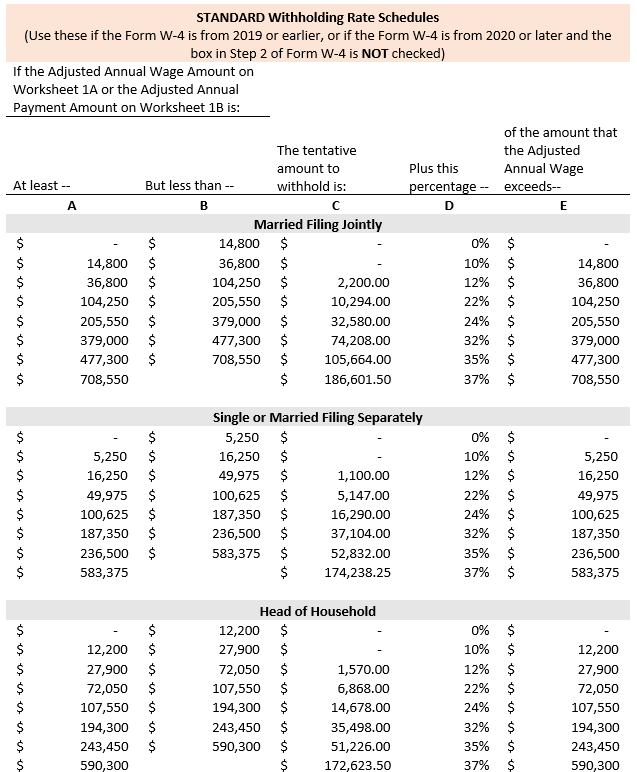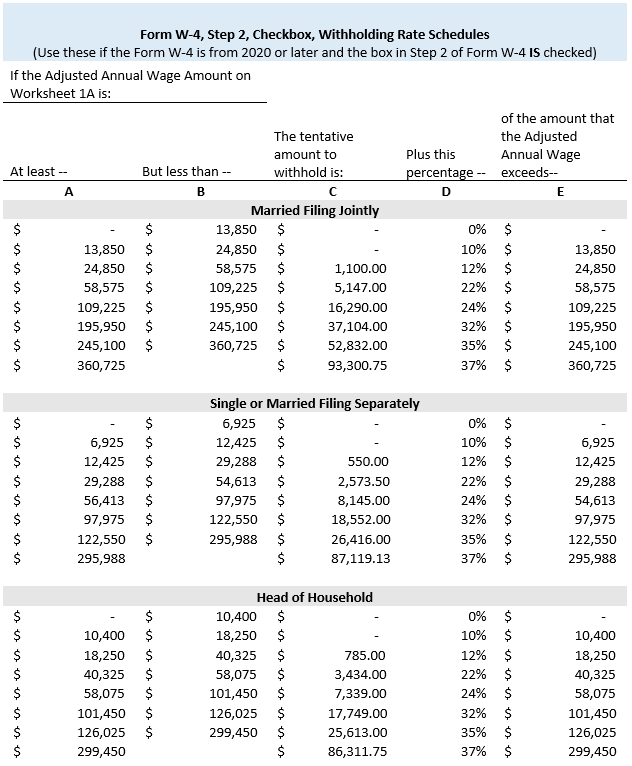# Calculation of Federal Employment Taxes

Calculation of Federal Employment Taxes

For Wages Paid on January 5, 2023, and Later

OASDI (Social Security) and Medicare Taxes

Wages for OASDI (Social Security) and Medicare are calculated by adding all earnings (including any taxable fringe benefits) less the following qualifying pre-tax deductions: insurance, parking, and UT FLEX. OASDI and Medicare taxes are calculated as follows:

• Find OASDI - Taxable Wages on the payslip, and multiply by 6.2 percent. The OASDI Taxable Wages have a wage limit of \$160,200 for the current tax year.
• Find Medicare - Taxable Wages on the payslip. Multiply by 1.45 percent on wages up to \$200,000. Multiply by 2.35 percent on wages above \$200,000.

Federal Withholding Taxes

Federal Withholding Taxable Wages are calculated by adding all earnings (including any taxable fringe benefits) less all pre-tax deductions, and less any applicable 1042-S Wages. The tax rate(s) used in the calculation are specific to earnings being paid.

Most payroll earnings use the IRS Percentage Method Tables for Income Tax; however, non-regular earnings (including, but not limited to: Awards, One-Time Merits, Relocation and Taxable Moving payments) use the Supplemental Wage flat rate of 22 percent.

IRS Percentage Method

To check your Federal Withholding Tax calculation for all earnings on the payslip, use the IRS Percentage Method below:

1. Find Federal Withholding – Taxable Wages in the Taxable Wages section of the payslip.
2. Annualize this amount based on the pay period frequency: If Monthly, multiply by 12; if Semi-Monthly, multiply by 24.
3. If you are a nonresident alien for tax purposes, with the exception of students from India, add:
1. \$9,550 if you are using a 2019 or earlier version of the W-4 Form.
2. \$13,850 if you are using a 2020 or later version of the W-4 Form.
4. Find the Withholding section on the payslip.
5. If you are using a 2019 or earlier version of the W-4 Form, follow these steps:
1. Subtract \$4,300 for each Allowance.
2. Find the Marital Status claimed, go to the Standard Withholding Rate Schedules (orange) IRS Percentage Method Table below, and use the appropriate status section of the table. Do not use the Head of Household table if the Form W-4 is from 2019 or earlier.
3. Find the row that your calculated amount falls in to. Take your calculated amount and:
1. Subtract the “of the amount that the Adjusted Annual Wage exceeds…” amount
2. Multiply by the percentage reflected in that row, and
3. Add the dollar amount reflected in “The tentative amount to withhold is…” column.
4. If you have a Monthly pay period frequency, divide by 12; if Semi-Monthly, divide by 24.
5. From the Withholding section of the payslip, if an Additional Withholding amount is reflected, add that amount to your calculated amount.
6. This should match the Federal Withholding amount in the Employee Taxes section of the payslip for this payment.
6. If you are using the 2020 or later version of the W-4 Form, follow these steps:
1. Add the amount listed as “Other Income” from the Withholding section of the payslip, if any.
2. Subtract the amount listed as “Deductions” from the Withholding section of the payslip, if any.
3. See if “Multiple Jobs or Spouse Works” from the Withholding section of the payslip is “Yes” or “No”.
1. If “Yes”, do not subtract any amount.
2. If “No” and your Marital Status is Married filing Jointly, subtract \$12,900.
3. If “No” and your Marital Status is any status other than Married filing Jointly, subtract \$8,600.
4. If the calculation is now a negative number, use \$0.00. Otherwise, continue using the amount you have calculated.
5. Find the row on the IRS Percentage Method Table below that your calculated amount falls into. Use the Standard Withholding Rate Schedules (orange) table if you selected “No” in Step 2 of the W-4 Form, “Multiple Jobs or Spouse Works”. Use the other (blue) table if you selected “Yes” in Step 2 of the W-4 Form, “Multiple Jobs or Spouse Works”.
1. Subtract the “of the amount that the Adjusted Annual Wage exceeds…” amount
2. Multiply by the percentage reflected in that row, and
3. Add the dollar amount reflected in “The tentative amount to withhold is…” column.
6. If you have a Monthly pay period frequency, divide by 12; if Semi-Monthly, divide by 24.
7. Divide the amount listed as “Total Dependent Amount” from the withholding section of the payslip, if any, by your pay period frequency (12 if Monthly, 24 if Semi-Monthly).
8. Subtract this de-annualized “Total Dependent Amount” from your calculated total from step f above. If this is now a negative number, use \$0.00. Otherwise, continue using the amount you have calculated.
9. Add the amount listed as “Additional Amount” from the Withholding section of the payslip, if any.
10. This should match the Federal Withholding amount in the Employee Taxes section of the payslip for this payment.Supplemental Wage Method

When a payslip includes recurring supplemental earnings (like a Faculty Endowed Supplement), these earnings are treated the same as regular salary and taxed based on the IRS Percentage method.

When a payslip includes only one-time supplemental earnings, multiply by 22 percent to calculate the Federal Withholding Tax amount for these earnings.

When a payslip includes earnings that are taxed using both methods (IRS Percentage and 22 percent one-time supplemental earnings), follow the steps below:

1. Categorize the earnings into both types, and total each category.
2. Allocate all the pre-tax deductions against both totals and subtract to arrive at two separate Federal Withholding – Taxable Wages amounts.
3. Multiply the supplemental earnings’ Federal Withholding – Taxable Wages by 22 percent.
4. Follow the IRS Percentage Method steps above to calculate the Federal Withholding Tax amount for the other earnings.
5. Add the two Federal Withholding Tax amounts together to match the total amount of Federal Withholding Taxes deducted on the payslip.

Last updated 01/09/2023